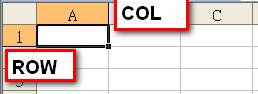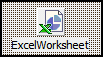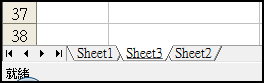## 2014/03/20

### TExcelWorksheet 取值ExcelWorksheet.Cells.Item[ROW, COL]

```//property Range[Cell1: OleVariant; Cell2: OleVariant]: ExcelRange read Get_Range;
ExcelWorksheet.Cells.Range['A1', EmptyParam].Value;
ExcelWorksheet.Cells.Range['A1',       'A1'].Value;
ExcelWorksheet.Cells.Range['A1', EmptyParam].Value2;
ExcelWorksheet.Cells.Range['A1',       'A1'].Value2;
```

ExcelWorksheet.UsedRange[_LCID].Columns.Count
ExcelWorksheet.UsedRange[_LCID].Rows.Count

## 2014/03/18

### ExcelXP TExcelWorksheet 連結 Worksheet

前情提要：
1.      使用 Delphi ExcelXP 元件開啟 Excel 和活頁簿(Workbook)
2.      把開啟的 Excel 和活頁簿關閉ExcelWorksheet 這個元件的連結方式比較特別，它必須：
1.      先指定前一節已開啟的ExcelWorkbook 指向哪一個 Worksheet

ExcelWorkbook.Worksheet[________]

這邊的填空處可以放兩種類型，一種是文字，另一種就是數字：
也就是 Worksheet 的「名字」或「排列的序號」以這個例子來說，我們要開啟「Sheet3」時，程式可以這麼寫：
ExcelWorkbook.Worksheet[Sheet3]

ExcelWorkbook.Worksheet
序號是按左至右，從 1 起始。

2.      ExcelWorksheet 元件連結或轉型後立即使用
I.            ExcelWorksheet 元件連結：(經常呼叫時使用)
ExcelWorksheet.ConnectTo(ExcelWorkbook.Worksheet[Sheet3] as _Worksheet);
II.         轉型後立即使用：(臨時呼叫時使用)
(ExcelWorkbook.Worksheet[Sheet3] as _Worksheet).ExcelWorksheet屬性

例如：
ExcelWorksheet.ConnectTo(ExcelWorkbook.Worksheet[Sheet3] as _Worksheet);
_YYYMM := ExcelWorksheet.Cells.Item[y, x];
_CONNO := ExcelWorksheet.Cells.Item[y1, x1];

_CURID := (ExcelWorkbook.Worksheet[Sheet3] as _Worksheet).Cells.Item[y1, x1]

不知道 Cells, Item 的屬性也沒有關係，下一節我們會再聊到。

## 2014/03/12

### 無狀態的DataSnap寫法參考

※※※ 已經 2014 年 4 月份完成 COM / RESTful 中間層的建置，目前看起來還是 COM 版的 DataSnap 效能要好。 ※※※

## 2014/03/10

### 悲劇的版本 Delphi 2009

(當時我以為 Delphi 2006 年版已經是爛中極致)